## Filters

Sort by :
Clear All
Q
Engineering
128 Views   |

A material  B  has twice the specific resistance of  A . A circular wire made of B  has twice the diameter of a wire made of A. Then for the two wires to   have the same resistance, the ratio     of their respective lengths must be

• Option 1)

• Option 2)

• Option 3)

• Option 4)

As we learnt in

- wherein

resistivity / specific resistance

Resistance of a wire

Option 1)

This option is correct.

Option 2)

This option is incorrect.

Option 3)

This option is incorrect.

Option 4)

This option is incorrect.

View More
Engineering
118 Views   |

The resistance of a bulb filament is    at a temperature of 100ºC. If its temperature coefficient of resistance be 0.005 per ºC   its resistance will become    at a temperature of

• Option 1)

• Option 2)

• Option 3)

• Option 4)

As we learnt in

Temperature co-efficient of Resistivity -

- wherein

Where the value of  is different at different temperatures

R100 =100

R = 200

= 0.005 C-1

Option 1)

This is incorrect.

Option 2)

This is incorrect.

Option 3)

This is correct.

Option 4)

This is incorrect.

View More
Engineering
1070 Views   |

The current  I   drawn from the 5  volt source will be :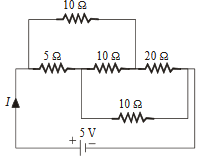• Option 1)

• Option 2)

• Option 3)

• Option 4)

As we learnt in

Wheat stone Bridge -

It is an arrangement of four resistances which can be used to measure one of them in terms of rest

- wherein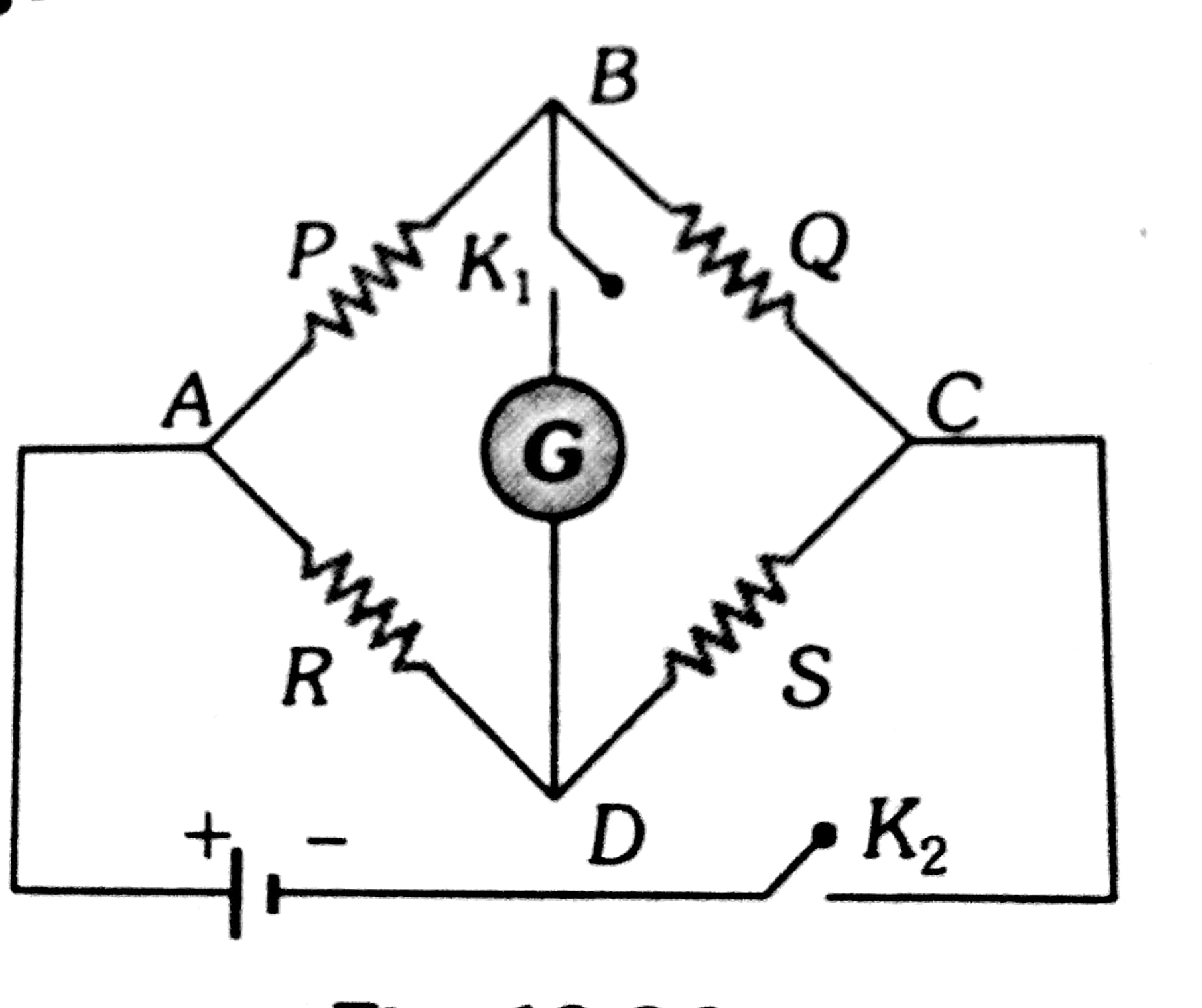The equivalent circuit is a balanced Wheatstone's bridge. Hence no current flows through arm BD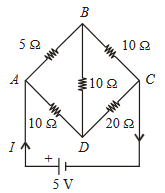AB and BC are in series

Option 1)

This option is incorrect.

Option 2)

This option is incorrect.

Option 3)

This option is correct.

Option 4)

This option is incorrect.

View More
Engineering
468 Views   |

In a Wheatstone’s bridge, three resistance P,Q  and R  connected in the three arms and the fourth arm is formed by two resistance S1 and S2  connected in parallel. The condition for bridge to be balanced will be

• Option 1)

• Option 2)

• Option 3)

• Option 4)

As we learnt in Wheat stone Bridge - It is an arrangement of four resistances which can be used to measure one of them in terms of rest - wherein    We know that    Option 1) This is incorrect. Option 2) This is incorrect. Option 3) This is correct. Option 4) This is incorrect.
Engineering
197 Views   |

The Kirchhoff’s first law   and second law    where the symbols have their usual meanings, are respectively based on

• Option 1)

conservation of charge, conservation of energy

• Option 2)

conservation of charge, conservation of momentum

• Option 3)

conservation of energy, conservation of charge

• Option 4)

conservation of momentum, conservation of charge.

As we learnt in

In closed loop -

- wherein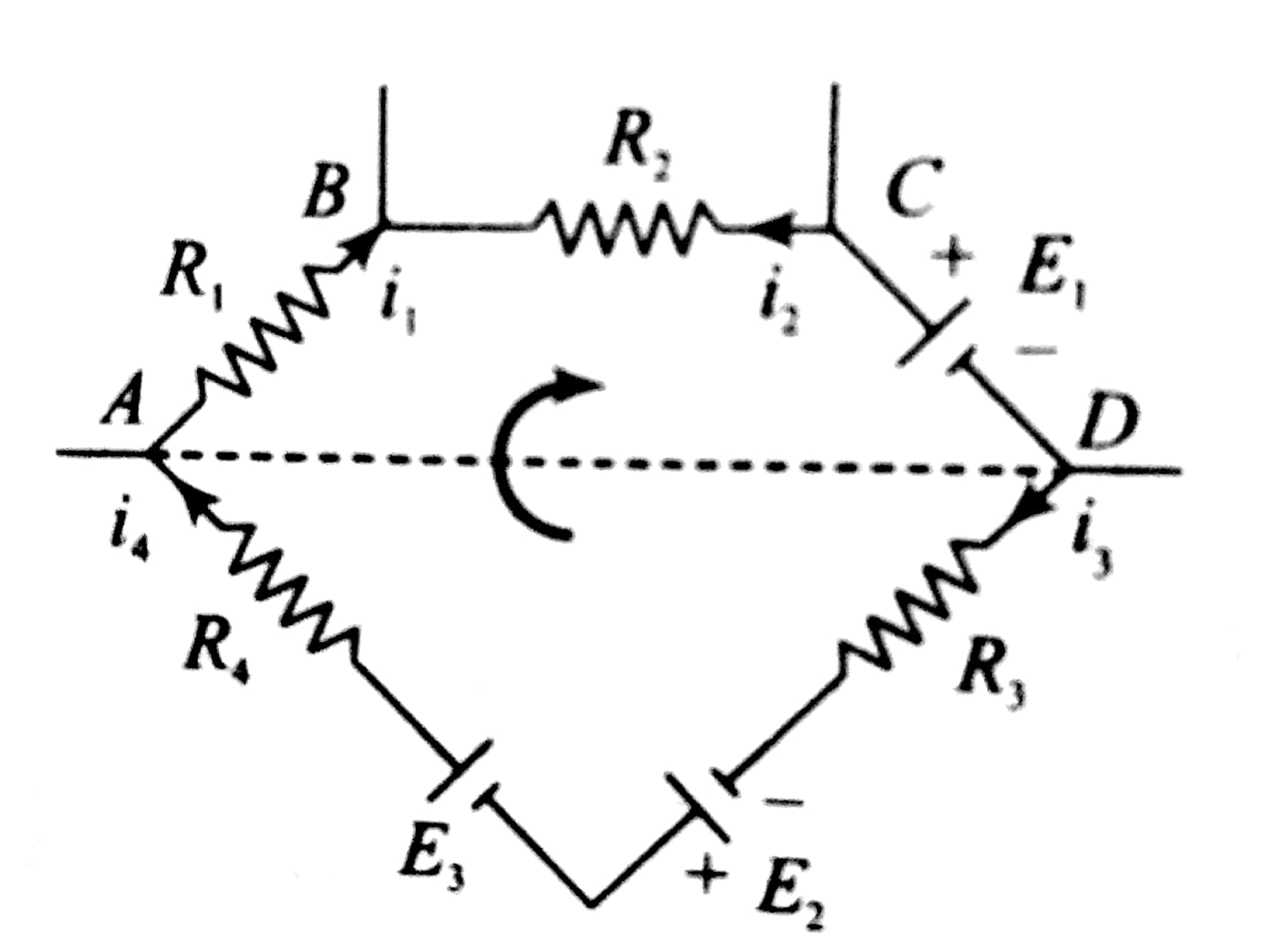and

Kirchoff's first law -

- wherein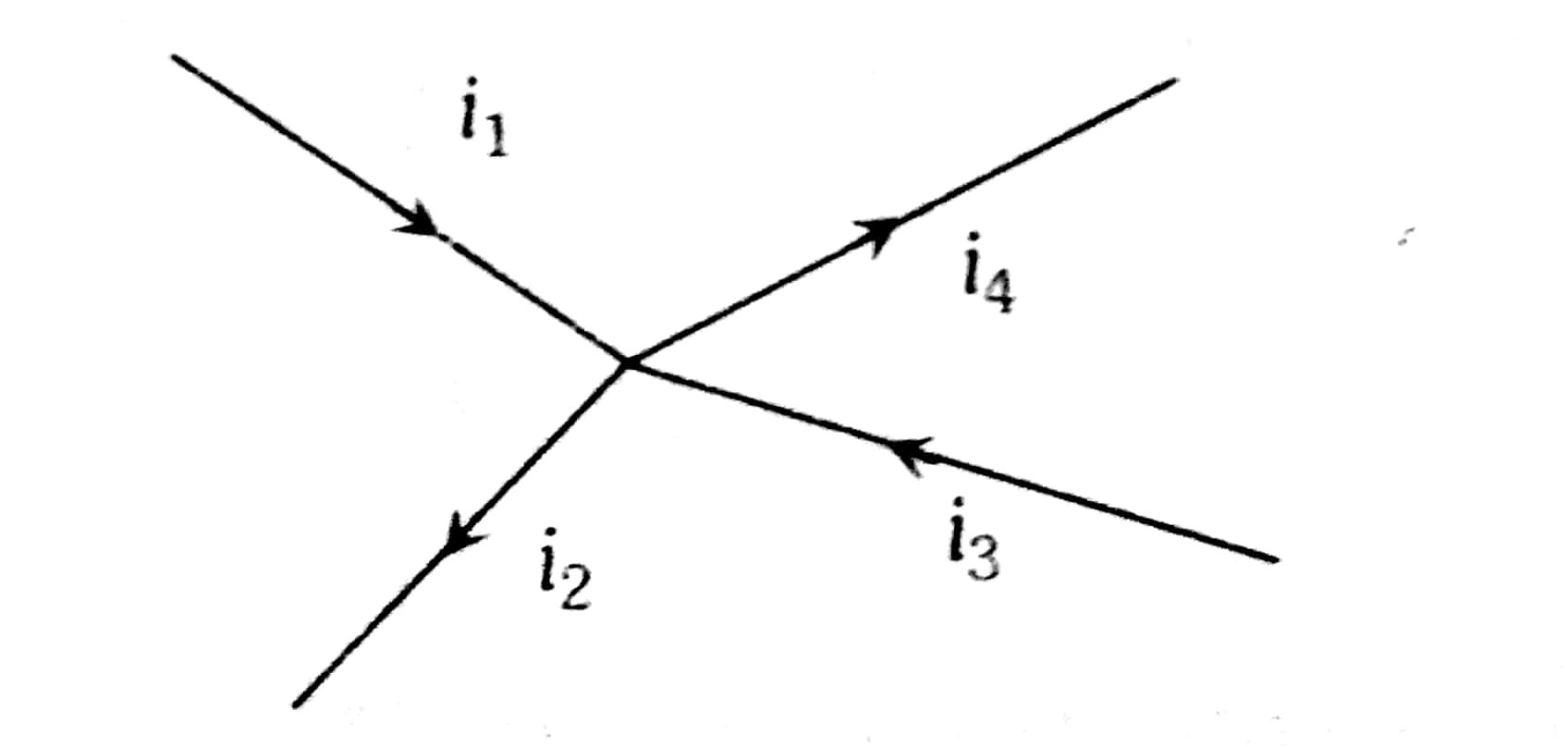Kirchhoff's first law      is based on conservation of charge

Kirchhoff's second law      is based on conservation of energy.

Option 1)

conservation of charge, conservation of energy

This is correct.

Option 2)

conservation of charge, conservation of momentum

This is incorrect.

Option 3)

conservation of energy, conservation of charge

This is incorrect.

Option 4)

conservation of momentum, conservation of charge.

This is incorrect.

View More
Engineering
550 Views   |

A thermocouple is made from two metals, antimony and bismuth. If one junction of the couple is kept hot and the other is kept cold then, an electric current will

• Option 1)

flow from antimony to bismuth at the cold junction

• Option 2)

flow from antimony to bismuth at the hot junction

• Option 3)

flow from bismuth to antimony at the cold junction

• Option 4)

not flow through the thermocouple.

As we learnt in In series Grouping - - wherein  Equivalent Resistance     At cold junction, current flow from Antimony to Bismuth (because current flows from metal occurring later in the series to metal occurring earlier in the thermoelectric series.   Option 1) flow from antimony to bismuth at the cold junction This option is correct. Option 2) flow from antimony to bismuth at the hot...
Engineering
286 Views   |

An electric bulb is rated 220 volt - ­ 100 watt. The power consumed by it when operated on 110 volt will be

• Option 1)

50 watt

• Option 2)

75 watt

• Option 3)

40 watt

• Option 4)

25 watt.

As we learnt in

if R=r -

- wherein

Power delivered will be maximum

Option 1)

50 watt

This option is incorrect.

Option 2)

75 watt

This option is incorrect.

Option 3)

40 watt

This option is incorrect.

Option 4)

25 watt.

This option is correct.

View More
Exams
Articles
Questions